Request a call back

# Class 9 NCERT Solutions Chemistry Chapter 3 - Atoms and Molecules

Study the law of conservation of mass from your textbook with supporting NCERT Solutions for CBSE Class 9 Chemistry Chapter 3 Atoms and Molecules. With TopperLearning’s expert solutions, you can learn to calculate molecular mass of oxygen, sulphur and other molecules.

Revise CBSE Class 9 Chemistry topics in the syllabus such as mole concept, polyatomic ions, atomic mass etc. using our textbook solutions. To ensure top performance in your exam, you need to practise these topics thoroughly. You can also use our revision notes, self-assessment tests and concept videos to achieve academic excellence.

## Atoms and Molecules Exercise 32

### Solution 1

The reactants in this reaction are sodium carbonate and ethanoic acid and the products are carbon dioxide, water and sodium ethanoate.
The total mass of the reactants = 5.3 + 6 = 11.3 g
The total mass of the products = 2.2 + 0.9 + 8.2 = 11.3 g
Thus, the total mass of the reactants is same as that of the products. Thus, the mass was neither created nor destroyed during the chemical reaction. Therefore, the mass was conserved during the reaction of sodium carbonate and ethanoic acid.

Concept insight: Firstly, recall the law of conservation of mass. And then check whether it is being followed by the data which is given or not.

## Atoms and Molecules Exercise 33

### Solution 2

The law of constant proportions states that in a chemical substance, the elements are always present in definite proportions by mass. Hydrogen and oxygen combine in the ratio of 1:8 by mass to form water.

1g of hydrogen reacts with oxygen = 8g

Thus, in order to form water, 3 x 8 = 24 g of oxygen will react completely with 3 g of hydrogen gas.

Concept insight: Recall the law of constant proportions and then apply that in a chemical substance, the elements are always present in definite proportions by mass.

### Solution 3

The second postulate of Dalton's atomic theory which states that "Atoms are indivisible particles, which cannot be created or destroyed in a chemical reaction" is the result of the law of conservation of mass.

Concept insight: For answering this question you should know both the law of conservation of mass and the postulates of Dalton's atomic theory.

### Solution 4

The postulate of Dalton's atomic theory which states that "The relative number and kinds of atoms are constant in a given compound" can explain the law of definite proportions.

Concept insight: For answering this question, you should know both law of definite proportions and the postulates of Dalton's atomic theory.

## Atoms and Molecules Exercise 35

### Solution 1

Atomic mass unit is a mass unit equal to exactly one-twelfth the mass of a carbon-12 atom. It is used to measure the masses of subatomic particles.

Concept insight: In this answer, write the proper definition of atomic mass unit.

### Solution 2

The dimension of atoms is very, very small and is of the order of 1 nm. Thus, we cannot see an atom with naked eyes.

Concept insight: For answering this question, you should recall the size of an atom.

## Atoms and Molecules Exercise 39

### Solution 1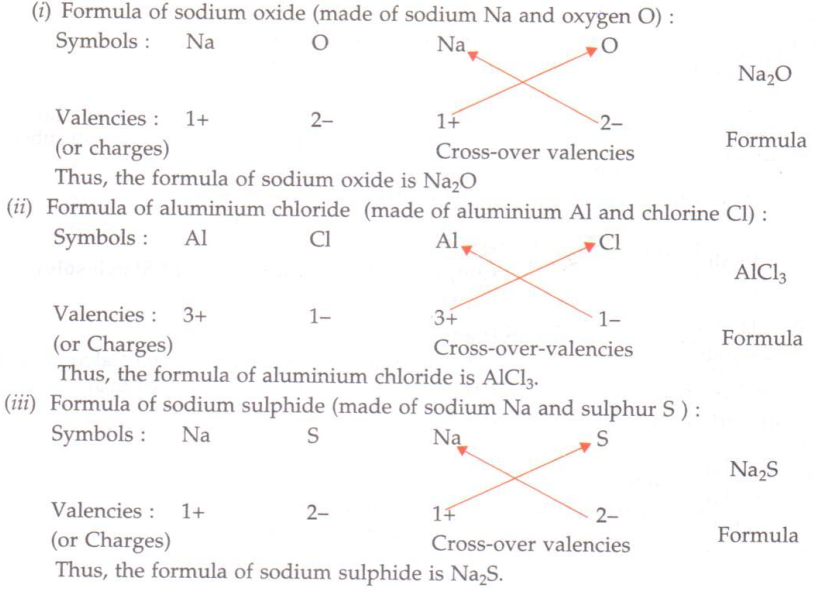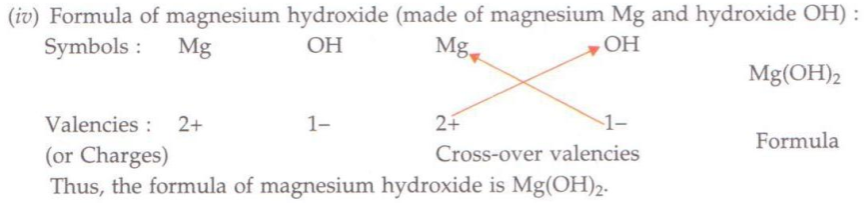Concept insight: For answering this question, write the cation and anion with the proper charges, cross multiply and write the formula.

### Solution 2

(i) Aluminium sulphate
(ii) Calcium chloride
(iii) Potassium sulphate
(iv) Potassium nitrate
(v) Calcium carbonate

Concept insight: For writing the name of the compound, write the name of the cation first and then the anion.

### Solution 3

The chemical formula of a compound is a symbolic representation of its composition.

Concept insight: For this question, write the proper definition of chemical formula.

### Solution 4

(i) Three (Two hydrogen atoms and one sulphur atom)
(ii) Five (One phosphorus and four oxygen)

Concept insight: Count the total number of atoms of each type and add them.

## Atoms and Molecules Exercise 40

### Solution 1

Molecular mass of H2 = Mass of 2H atoms = 1u + 1u = 2u
Molecular mass of O2 = Mass of 2O atoms = 16u + 16u = 32u
Molecular mass of Cl2 = Mass of 2Cl atoms = 35.5u + 35.5u = 71u
Molecular mass of CO2 = Mass of C atom + 2 x Mass of O atom = 12u + (2 16u) = 44u
Molecular mass of CH4 = Mass of C atom + 4 x Mass of H atom = 12u + (4 1 u) = 16u
Molecular mass of NH3 = Mass of N atom + 3 x Mass of H atom = 14u + (3 1 u) = 17u
Molecular mass of CH3OH = Mass of C atom + 3 x Mass of H atom + Mass of O atom + Mass of H atom = 12u + (3 1 u) + 16 u + 1 u = 32u

Concept insight: For calculating the molecular mass, add the atomic masses of all the atoms present in the formula.

### Solution 2

Formula unit mass of ZnO = 65 u + 16 u = 81 u
Formula unit mass of Na2O = (2 x 23 u) + 16 u = 62 u
Formula unit mass of K2CO3 = (2 x 39 u) + 12 u + (3 x 16 u) = 138 u

Concept insight: For calculating the formula unit mass, add the atomic masses of all the atoms present in the formula.

## Atoms and Molecules Exercise 42

### Solution 1

1 mole = 6.022 x 1023 atoms
One mole of carbon atoms weighs 12 gram.

i.e. 6.022 x 1023 atoms of carbon weigh 12 gram.

Therefore,
1 atom of carbon weighs 12 / (6.022 x 1023) = 1.993 x 10-23 gram.

Concept insight: For answering questions related to mole concept apply the relation### Solution 2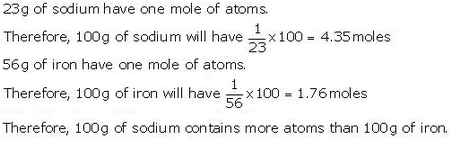Concept insight: For answering questions related to mole concept, apply the relation## Atoms and Molecules Exercise 43

### Solution 1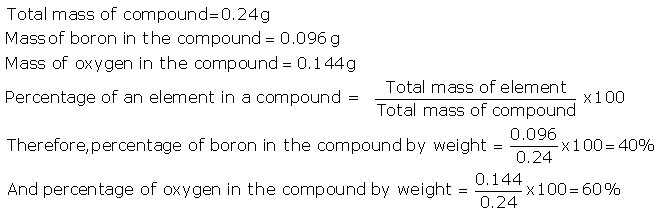Concept insight:

Always remember,### Solution 2

3.0 g of carbon combines with 8.0 g of oxygen to give 11.0 of carbon dioxide.
Thus, by the law of definite proportions, when 3.00 g of carbon is burnt in 50.00 g of oxygen, only 8.00 g of oxygen will be used to produce 11.00 gram of carbon dioxide. The remaining 42.00 g of oxygen will remain unreacted. Law of constant proportion is governed.

Concept insight: Relate the given data in the question to the laws and then identify which law is governing the answer.

## Atoms and Molecules Exercise 44

### Solution 3

Polyatomic ions are those ions that are formed from groups of joined atoms. These atoms can be of the same type or of different type. Some examples of polyatomic ions are NO3-, NH4+, OH-, SO42-, and SO32-.

Concept insight: Recall the definition of polyatomic ions and give examples of it. It is an important question from exam point of view.

### Solution 4

(a) MgCl2
(b) CaO
(c) Cu(NO3)2
(d) AlCl3
(e) CaCO3

Concept insight: For answering this question, write the cation and anion with the proper charges, cross multiply and write the formula.

### Solution 5

(a) Calcium and oxygen
(b) Hydrogen and bromine
(c) Sodium, hydrogen, carbon, and oxygen
(d) Potassium, sulphur, and oxygen

Concept insight: For answering this question, write the formula of the compound first, see which all atoms are present in it and then write their names.

### Solution 6

(a) Molecular mass of ethyne, C2H2 = 2 x Mass of C + 2 x Mass of H = 212u + 2u = 26u
(b) Molecular mass of sulphur molecule, S8 = 8 x Mass of S = 832u = 256u
(c) Molecular mass of phosphorus molecule, P4 = 4 x Mass of P = 431u = 124u
(d) Molecular mass of HCl = Mass of H + Mass of Cl = 1 u + 35.5u = 36.5u
(e) Molecular mass of HNO3 = Mass of H + Mass of N + 3 x Mass of O = 1 u + 14u + 316u = 63u

Concept insight: For calculating the molecular mass, add the atomic masses of all the atoms present in the formula.

### Solution 7

(a) Mass of one mole of nitrogen atoms = molecular mass of nitrogen atoms in grams = 14 g
(b) Mass of 4 moles of aluminium atoms = 4 x Mass of 1 mole of Al atoms = 4 x molecular mass of aluminum atoms in grams = 4 x 27 = 108 g
(c) Mass of 10 moles of sodium sulphite = 10 x Mass of 1 mole of Na2SO3 = 10 x molecular mass of Na2SO3 in grams
Molecular mass of sodium sulphite, Na2SO3 = 2 x 23 + 32 + 3 x 16 = 126 g
Thus, the mass of 10 moles of sodium sulphite = 10 x 126 = 1260 g

Concept insight: For answering questions related to mole concept, apply the relation

For an atom ,
1 mole =  Gram atomic mass = 6.0221023 atoms
For a molecule,
1 mole = Gram atomic mass = 6.0221023 molecules

### Solution 8Concept insight: For answering questions related to mole concept, apply the relation:### Solution 9

(a) The mass of 1 mole of oxygen atoms = 16 g
Thus, the mass of 0.2 mole of oxygen atoms = 160.2 = 3.2 g
(b) The mass of 1 mole of water molecules = 18 g
Thus, the mass of 0.5 mole of water molecules = 180.5 = 9.0 g

Concept insight: For answering questions related to mole concept, apply the relation:

For  an atom,
1 mole = Gram atomic mass = 6.0221023
For  a molecule,
1 mole = Gram molecule mass = 6.0221023

### Solution 10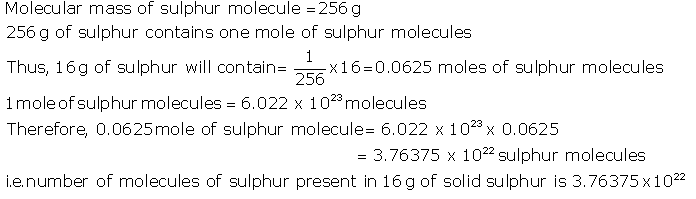Concept insight: For answering questions related to mole concept, apply the relation:

For  an atom,
1 mole = Gram atomic mass = 6.0221023
For  a molecule,
1 mole = Gram molecule mass = 6.0221023

### Solution 11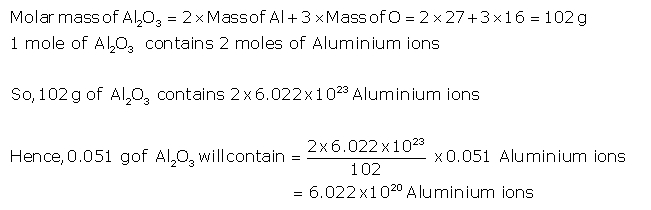Concept insight: For answering questions related to mole concept, apply the relation:

For  an atom,
1 mole = Gram atomic mass = 6.0221023
For  a molecule,
1 mole = Gram molecule mass = 6.0221023